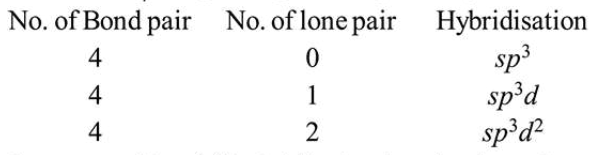# If $mathrm{AB}_{4}$ molecule is a polar molecule,

Question:

If $\mathrm{AB}_{4}$ molecule is a polar molecule, a possible geometry of $\mathrm{AB}_{4}$ is :

1. Square pyramidal

2. Tetrahedral

3. Rectangular planar

4. Square planar

Correct Option: 1

Solution:

For $\mathrm{AB}_{4}$ compound possible geometry areStructure with $s p^{3} d^{2}$ hybridisation is polar due to lone pair moment while in other possibilities molecules is non-polar. Square pyramidal can be polar due to lone pair moment as the bond pair moments will get cancelled out.